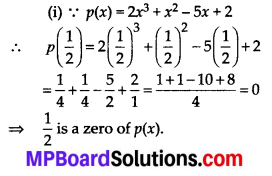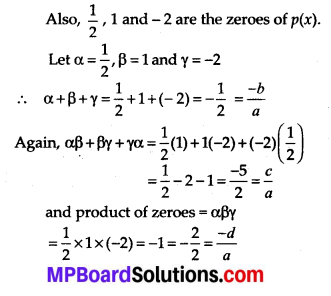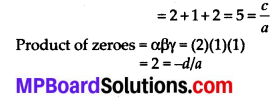# MP Board Class 10th Maths Solutions Chapter 2 Polynomials Ex 2.4

In this article, we will share MP Board Class 10th Maths Book Solutions Chapter 2 Polynomials Ex 2.4 Pdf, These solutions are solved subject experts from the latest edition books.

## MP Board Class 10th Maths Solutions Chapter 2 Polynomials Ex 2.4

Question 1.
Verify that the numbers given alongside of the cubic polynomials below are their zeroes. Also verify the relationship between the zeroes and the coefficients in each case:
(i) 2x3 + x2 – 5x + 2; $$\frac{1}{2}$$, 1, -2
(ii) x3 – 4x2 + 5x – 2; 2, 1, 1
Solution:Again, p(1) = 2(1)3 + (1)2 – 5(1) + 2
= 2 + 1 – 5 + 2 = 0
⇒ 1 is a zero of p(x).
Also, p(-2) = 2(-2)3 + (-2)2 – 5(-2) + 2
= -16 + 4 + 10 + 2 = -16 +16 = 0
= -2 is a zero of p(x).
Now, p(x) = 2x3 + x2 – 5x + 2
∴ Comparing it with ax3 + bx2 + cx + d, we have a = 2, b = 1, c = -5, and d = 2Thus, the relationship between the coefficients and the zeroes of p(x) is verified.

(ii) Here, p(x) = x3 – 4x2 + 5x – 2
∴ p(2) = (2)3 – 4(2)2 + 5(2) – 2
= 8 – 16 + 10 – 2 = 18 – 18 = 0
⇒ 2 is a zero of p(x)
Again p(1) = (1)3 – 4(1)2 + 5(1) – 2
= 1 – 4 + 5 – 2 = 6 – 6 = 0
⇒ 1 is a zero of p(x)
Now, comparing p(x) = x3 – 4x2 + 5x – 2
with ax3 + bx2 + cx + d = 0, we have
a = 1, b = -4, c = 5 and d = -2
Also 2, 1 and 1 are the zeroes of p(x).
Let α = 2,
β = 1,
γ = 1
Now, sum of zeroes = α + β + γ
= 2 + 1 + 1 = 4 = -b/aThus, the relationship between the zeroes and the coefficients of p(x) is verified.Question 2.
Find a cubic polynomial with the sum, sum of the product of its zeroes taken two at a time, and the product of its zeroes as 2, -7, -14 respectively.
Solution:
Let the required cubic polynomial be ax3 + 6x2 + cx + d = 0 and its zeroes be α, β and γ.∴ The requied cubic polynomial is
1x3 + (-2)x2 + (-7)x + 14 = 0
= x3 – 2x2 – 7x + 14 = 0Question 3.
If the zeroes of the polynomial x3 – 3x2 + x + 1 are a -b, a, a + b, find a and b.
Solution:
We have p(x) = x3 – 3x2 + x + 1
Comparing it with Ax3 + Bx2 + Cx + D,
We have A = 1, B = -3, C = 1 and D = 1
∵ It is given (a – b), a and (a + b) are the zeroes of the polynomial.Question 4.
If two zeroes of the polynomial
x4 – 6x3 – 26x2+ 138x – 35 are 2±$$\sqrt{3}$$, find other zeroes.
Solution:
Here, p(x) = x4 – 6x3 – 26x3 + 138x – 35
∵ Two of the zeroes of p(x) are : 2 ± $$\sqrt{3}$$(x2 – 4x + 1)(x2 – 2x – 35) = p(x)
⇒ (x2 – 4x + 1) (x2 – 7x + 5x – 35) = p(x)
⇒ (x2 – 4x + 1) [x(x – 7) + 5(x – 7)] = p(x)
⇒ (x2 – 4x + 1)(x – 7)(x + 5) = p(x)
i.e., (x – 7) and (x + 5) are other factors of p(x).
∴ 7 and – 5 are other zeroes of the given polynomial.Question 5.
If the polynomial x4 – 6x3 + 16x2 – 25x + 10 is divided by another polynomial x2 – 2x + k, the remainder comes out to be x + a, find k and a.
Solution:
Applying the division algorithm to the polynomials x4 – 6x3 + 16x2 – 25x + 10 and x2 – 2x + k, we have∴ Remainder = (2k – 9)x – k(8 – k) + 10
But the remainder = x + a (Given)
Therefore, comparing them, we haveand a = -k(8 – k) + 10
= -5(8 – 5) + 10
= -5(3) + 10 = -15 + 10 = -5
Thus k = 5 and a = -5